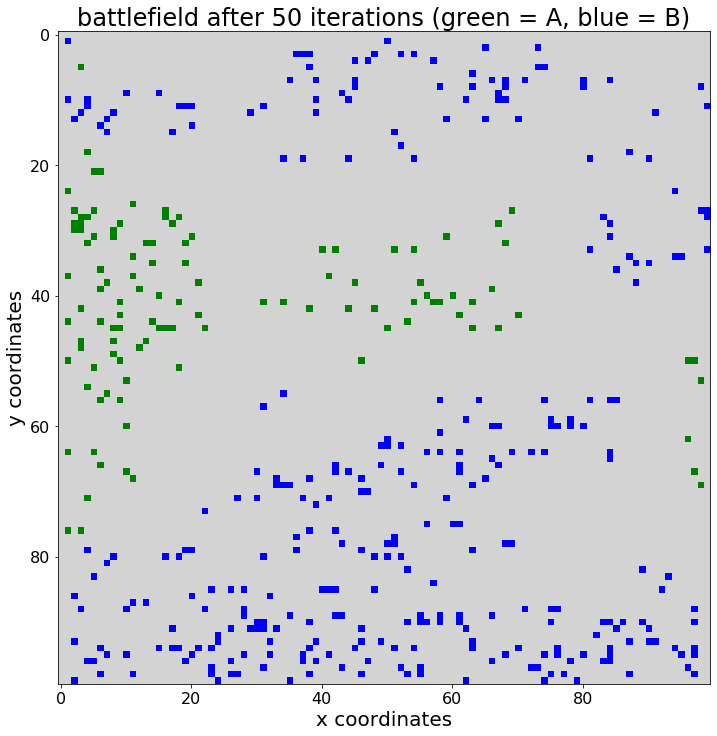# 一个简单的基于代理的模拟运行,使用Python中的Matplotlib进行可视化```# 类，将代理定义为抽象数据类型
class agent:
#  代理的构造方法
def __init__(self,x,y,group):
self.life = 100 # 特工的生活得分
self.x = x
self.y = y
self.group = group```

```# 在python中使用列表推导创建空的100 x 100列表
battlefield = [[None for i in range(0,100)] for i in range(0,100)]```

```# -- 定义用于创建代理并将其分配到网格的功能
def agentCreator(size,group,groupList,field,n,m):
# 遍历整个组，即1000个单位
for j in range(0,size):
# 选择随机可用位置
while True:
# 随机x坐标e
x = random.choice(range(0,n))
# 随机y坐标
y = random.choice(range(0,m))
# 检查现货是否可用；如果没有，请再次重申
if field[x][y] == None:
field[x][y] = agent(x=x,y=y,group=group)
# 将代理对象引用附加到组列表
groupList.append(field[x][y])
# 退出while循环；现场拍摄
break```

```列出可用的x和y位置
locations = battlefield.copy() # 使用.copy防止通过引用复制
# 为将来创建包含代理引用的空列表，键入A和B
agents_A = []
agents_B = []
# 为A和B组的特工分配随机点；
import random
agentCreator(size = 1000,
group = "A",
groupList = agents_A,
field = battlefield,
n = 100,
m = 100)
agentCreator(size = 1000,
group = "B",
groupList = agents_B,
field = battlefield,
n = 100,
m = 100)
# .imshow（）需要一个带有float元素的矩阵；
population = [[0.0 for i in range(0,100)] for i in range(0,100)]
# 如果座席类型为A，则放置1.0；如果座席类型为B，则放置2.0
for i in range(1,100):
for j in range(1,100):
if battlefield[i][j] == None: # 空
pass # 在总体单元格中保留0.0
elif battlefield[i][j].group == "A": # A组代理商
population[i][j] = 1.0 # 1.0表示“ A”
else: # B组代理商
population[i][j] = 2.0 # 2.0表示“ B”
# 从matplotlib导入pyplot和颜色
from matplotlib import pyplot, colors
# 使用matplotlib中的颜色，定义颜色图
colormap = colors.ListedColormap(["lightgrey","green","blue"])
# 使用pyplot定义图形大小
pyplot.figure(figsize = (12,12))
# 使用pyplot添加标题
pyplot.title("battlefield before simulation run (green = A, blue = B)",
fontsize = 24)
# 使用pyplot添加x和y标签
pyplot.xlabel("x coordinates", fontsize = 20)
pyplot.ylabel("y coordinates", fontsize = 20)
# 使用pyplot调整x和y轴刻度
pyplot.xticks(fontsize = 16)
pyplot.yticks(fontsize = 16)
# 使用pyplot中的.imshow（）方法可视化代理位置
pyplot.imshow(X = population,
cmap = colormap)
```
`<matplotlib.image.AxesImage at 0x22495922ac8>`

A组的策略是始终在每一轮中都击中相同的特工

B组有随机且独立的攻击敌人的策略。这意味着每个B型特工都将在该特工触及的范围内攻击随机选择的敌人。

1）每轮是一次迭代

2）在每个回合中，每个特工都可以攻击他所能到达的一个特工

3) 代理的范围是在模拟开始时定义的，默认为10

4) 如果特工死亡，他将不再位于战场上

5) 特工的生活得分等于或低于零时会死亡

6) 每个特工都有一个随机分布的攻击力，范围从10到60

7) 在每个回合中，所有特工都会发动攻击

```for counter in range(0,50): # 在这种情况下，我要进行10次迭代
# 遍历战场上的所有细胞
for i in range(0,len(battlefield)):
for j in range(0,len(battlefield))
# 检查相应单元格中是否有代理
if battlefield[i][j] != None:
# 根据类型：执行相应的攻击策略
if battlefield[i][j].group == "A":
found_i = None
found_j = None
# 每次迭代以相同顺序查看邻居单元
for k in range(i-10,i+11):
for l in range(j-10,j+11):
# 检查负索引值；如果是这样-打破！
if k < 0 or l < 0:
break
# 检查索引值是否大于99，如果这样的话，请中断！
if k > 99 or l > 99:
break
if battlefield[k][l]:
if battlefield[k][l].group == "B": # 那是一个敌人
if found_i == None:
found_i = k
found_j = l
# 对已识别的敌人造成伤害
if found_i:
battlefield[found_i][found_j].life = battlefield[found_i][found_j].life - random.randint(10,60)
else:
# 首先检查周围的牢房中是否有一个敌人
enemy_found = False
for k in range(i-10,i+11):
for l in range(j-10,j+11):
# 检查负索引，如果是，则中断下一次迭代
if k < 0 or l < 0:
break
# 检查索引值是否大于99（如果是）
if k > 99 or l > 99:
break
if battlefield[k][l] != None:
if battlefield[k][l].group == "A":
enemy_found = True
# 选择一个随机行和一个随机列
found_i = None
found_j = None
while enemy_found and found_i == None:
k = random.randint(i-10,i+10)
l = random.randint(j-10,j+10)
# 检查负索引，如果是，则继续下一个迭代
if k < 0 or l < 0:
continue
# 检查索引值> 99，如果是，则继续
if k > 99 or l > 99:
continue
if k != i:
if battlefield[k][l]:
if battlefield[k][l].group == "A":
found_i = k
found_j = l
else:
if l != j:
if battlefield[k][l]:
if battlefield[k][l].group == "A":
found_i = k
found_j = l
# 对已识别的敌人造成伤害
if found_i:
battlefield[found_i][found_j].life = battlefield[found_i][found_j].life - random.randint(10,60)
# 识别生活得分为或低于分数的代理商-并将其从网格中删除
for i in range(0,len(battlefield)):
for j in range(0,len(battlefield)):
if battlefield[i][j]:
if battlefield[i][j].life <= 0:
battlefield[i][j] = None
# 生成所有战场位置的图，此后10次迭代
population = [[0.0 for i in range(0,100)] for i in range(0,100)]
# 如果agent是A类型，则输入1.0；如果是B类型，则pyt 2.0
for i in range(1,100):
for j in range(1,100):
if battlefield[i][j] == None: # 空的
pass # 在总体单元格中保留0.0
elif battlefield[i][j].group == "A": # A组代理商
population[i][j] = 1.0 # 1.0表示“ A”
else: # B组代理商
population[i][j] = 2.0 # 2.0表示“ B”
# 从matplotlib导入pyplot和颜色
from matplotlib import pyplot, colors
# 使用matplotlib中的颜色，定义颜色图
colormap = colors.ListedColormap(["lightgrey","green","blue"])
# 使用pyplot定义图形大小
pyplot.figure(figsize = (12,12))
# 使用pyplot添加标题
pyplot.title("battlefield after 50 iterations (green = A, blue = B)",
fontsize = 24)
# 使用pyplot添加x和y标签
pyplot.xlabel("x coordinates", fontsize = 20)
pyplot.ylabel("y coordinates", fontsize = 20)
# 使用pyplot调整x和y轴刻度
pyplot.xticks(fontsize = 16)
pyplot.yticks(fontsize = 16)
# 使用pyplot中的.imshow（）方法可视化代理位置
pyplot.imshow(X = population,
cmap = colormap)```
`<matplotlib.image.AxesImage at 0x22495be1848>`